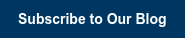TALK TO A PERSON: 800.321.3526 | AGENCY: 888.240.3351
BlogIf you’re borrowing money, interest is the cost you pay to borrow. In the case of investment, interest is a way invested funds can generate a return for the investor. Interest is expressed as a percentage, but to understand the amount of interest that you will be charged for a loan or earn on an investment or another financial product, you have to look beyond this number to whether the interest is calculated as a simple or compound rate. If two accounts both have an interest rate of 5%, for example, but one is simple and the other is compound, they will accrue very different amounts of interest over time.

## Simple Interest

As the name suggests, simple interest is the easiest to understand. It’s most commonly used for bonds, short-term loans, and open-ended borrowing arrangements, such as credit cards. To calculate simple interest, the percentage rate is applied to the initial amount borrowed or invested and multiplied by the applicable number of years.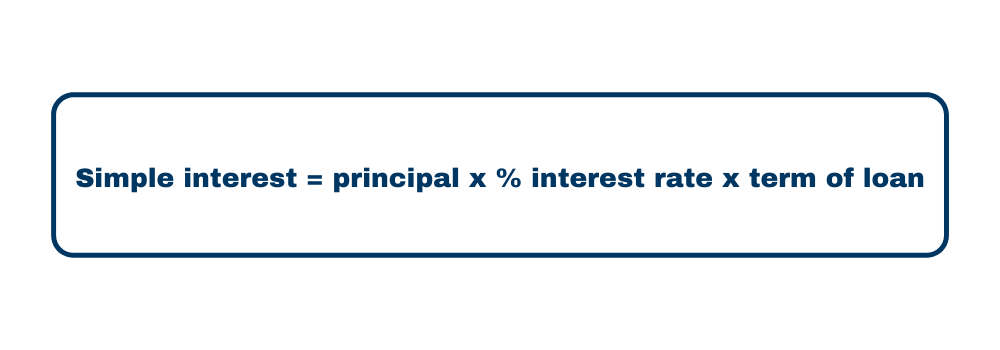For example, if you were to invest 10,000 in an account with a simple interest rate of 5% for five years, that initial \$10,000 would earn \$2,500. If you kept the investment for 10 years, the rate of return would remain constant, amounting to \$5,000 in the end.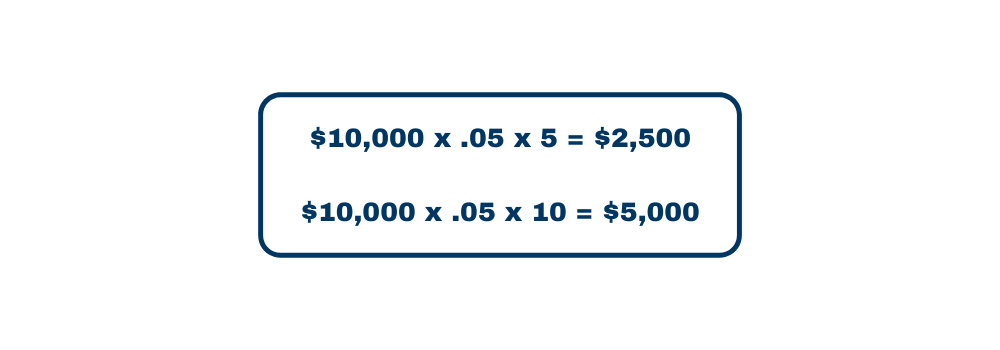## Compound Interest

Compound interest is more commonly used for loans and investments designed to be in effect for several years. Compound interest accumulates faster than simple interest because it accrues not only on the initial principal amount but also on interest that has already been earned. As a result, the formula is a bit more complex: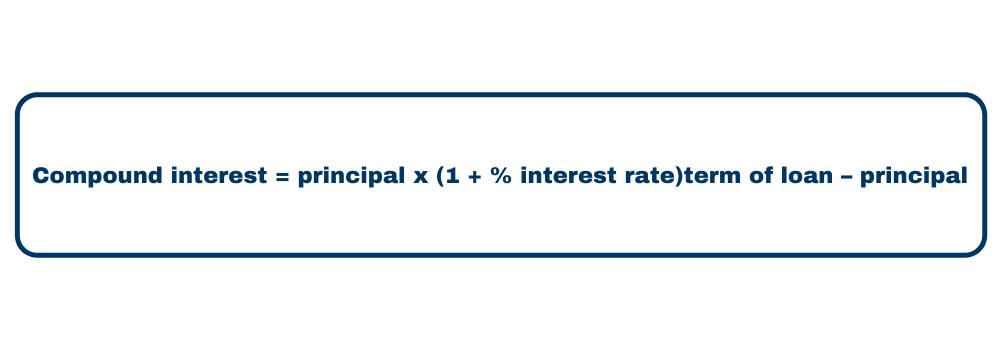In short, the amount upon which interest accumulation is based is always the principal of the account when using simple interest, while the total account value is used when calculating compound interest. To apply the example above, if your 10,000 investment were to earn compound interest at a rate of 5% per year for five years, your gain would be \$2,762.82. With compound interest, growth continues to accelerate so that after ten years, earnings would reach \$6,288.95.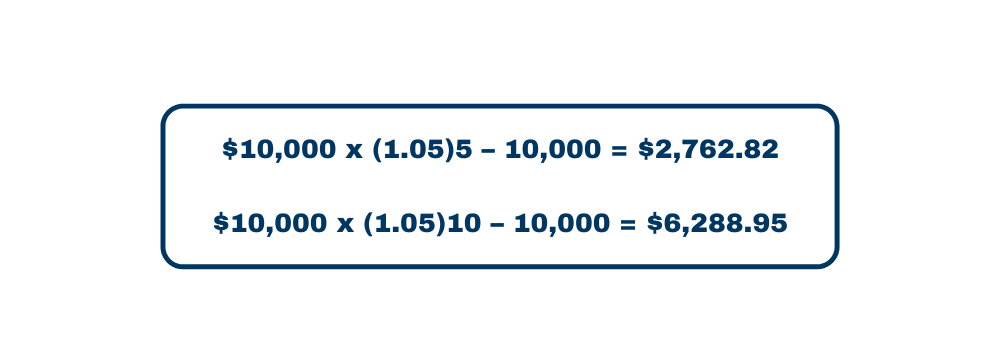As you can see, compound interest gives your money the best opportunity to grow when you’re investing—and costs more when you’re borrowing. If you’re looking to create financial stability for your retirement years, compound interest will let your money take you farther.

## Compound Growth for Secure Retirement

Annuities are financial products that use an initial contribution to build value over time. ELCO’s Guardian Eagle Annuity pays compound interest at a rate that varies with the terms of the account. The annuity’s value grows tax deferred, so you won’t pay taxes on the earnings until you withdraw them. A variety of terms lets you choose the guaranteed period and corresponding interest rate that best suits your financial needs, and generous liquidity options allow free access to a portion of the principal once per year (beginning in the second contract year) to give you the flexibility you need to keep up with changing financial circumstances.